# Solve for the component forces using the method of Pins. bo 124 103.727 5).037 1 b...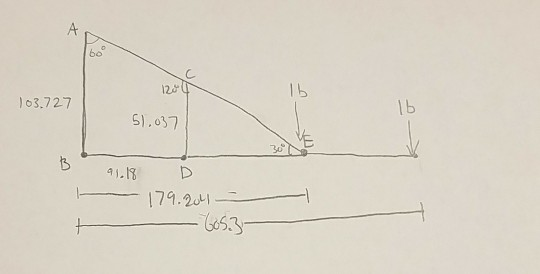Solve for the component forces using the method of Pins.

bo 124 103.727 5).037 1 b 30 41.13 D 179.201 !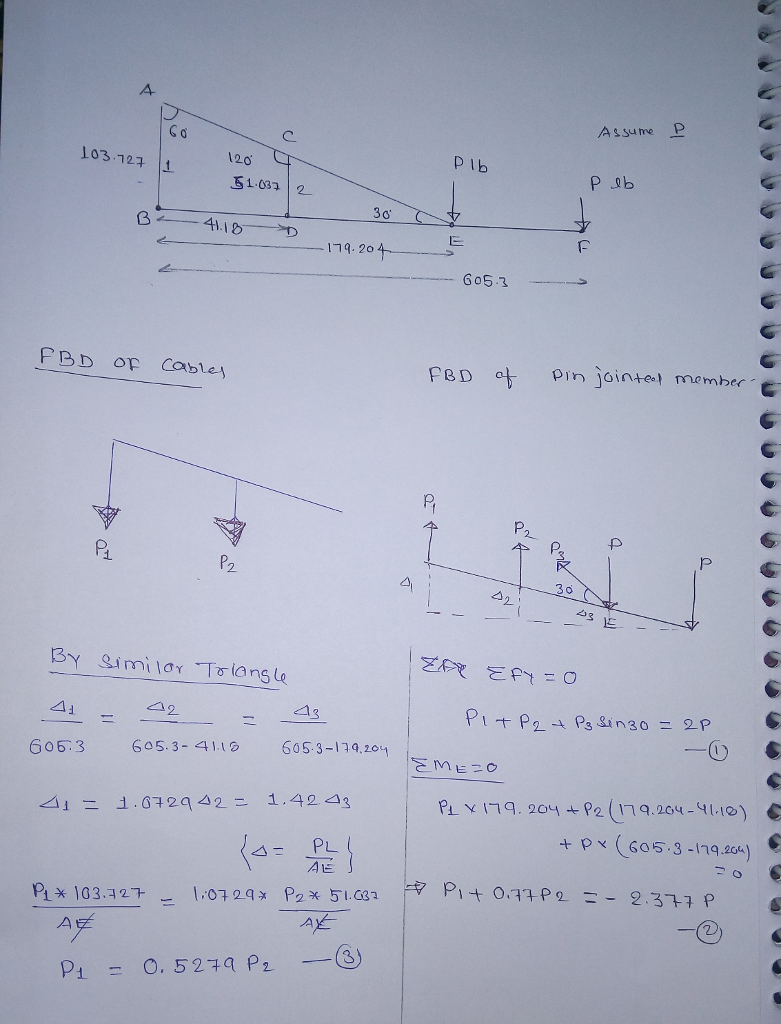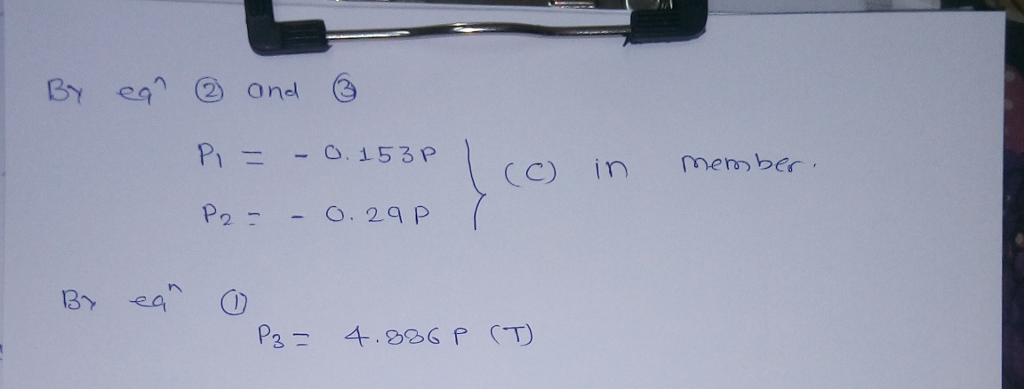#### Earn Coin

Coins can be redeemed for fabulous gifts.

Similar Homework Help Questions
• ### ASSIGNMENT 1 1. Determine the resultant of the forces using the component method. P 20N 42...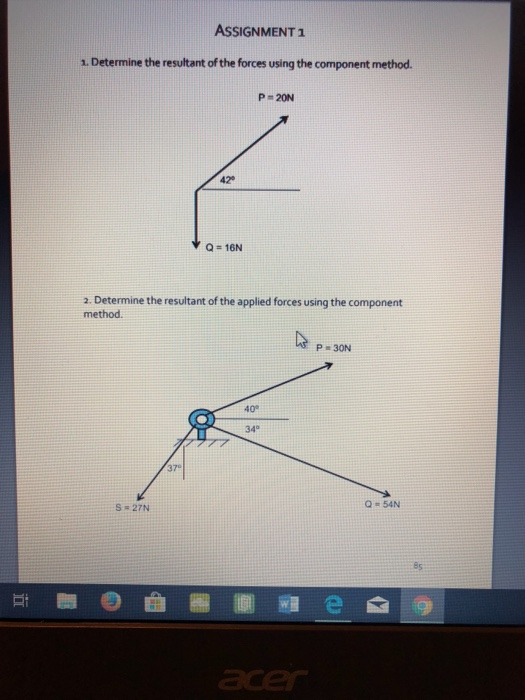ASSIGNMENT 1 1. Determine the resultant of the forces using the component method. P 20N 42 2. Determine the resultant of the applied forces using the component method. P-30N 40° 34° 37 Q = 54N S 27N 8s

• ### Determine the resultant R of the forces below. Solve using the tabular methodor using the graphical method showing magnitude (2 dec. places) and resultant angle (1 dec. place). 1.) Determine...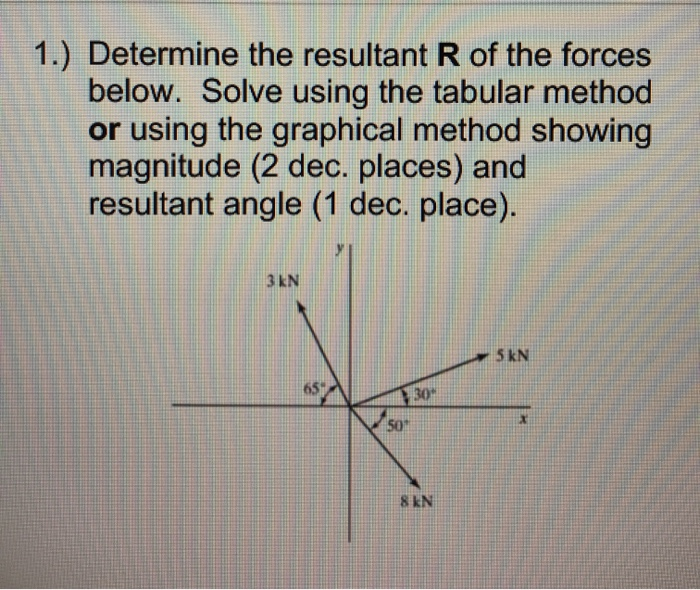Determine the resultant R of the forces below. Solve using the tabular methodor using the graphical method showing magnitude (2 dec. places) and resultant angle (1 dec. place). 1.) Determine the resultant R of the forces below. Solve using the tabular method or using the graphical method showing magnitude (2 dec. places) and resultant angle (1 dec. place). 3 kN 5 kN 30% 507 8 kN 1.) Determine the resultant R of the forces below. Solve using the tabular method...

• ### need complete answer please 2- Find the resultant of the following two forces using component method:...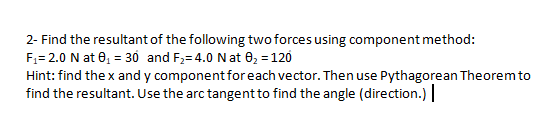need complete answer please 2- Find the resultant of the following two forces using component method: Fi= 2.0 N at 01 = 30 and F2=4.0 N at O2 = 120 Hint: find the x and y component for each vector. Then use Pythagorean Theorem to find the resultant. Use the arc tangent to find the angle (direction.) |

• ### Module -9. Test Q. 1 Determine the resultant forces at pins B and C on member...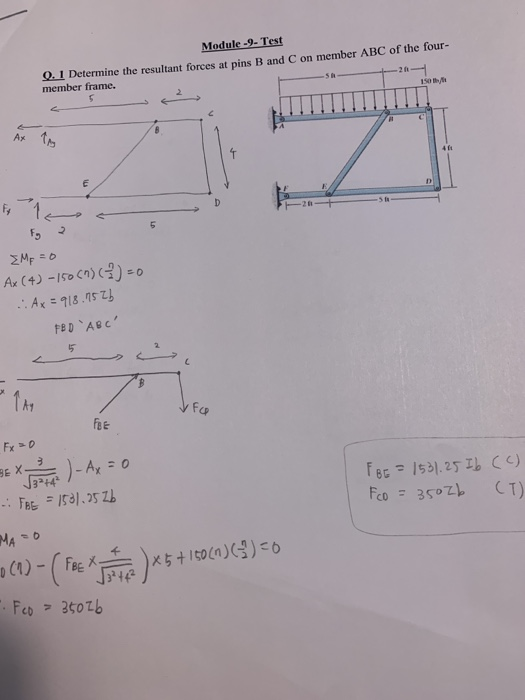Module -9. Test Q. 1 Determine the resultant forces at pins B and C on member ABC of the four- member frame. 5 8 Ax to 411 E F9 ΣΜΕ εο Ax (4) -150 (1) (-1) = 0 ..Ax = 918.052b FED 'ABC' 15 Fa FEE 3-14 FBE = 1531.2526 F BE = 1531.25 Ib (c) Fco = 35026 (T) MAO (0)-(Fra) ***120)(2)- -. Feo - 36026

• ### Solve All joints and supports using moment distribution method! Problem 2. Solve the moments at all...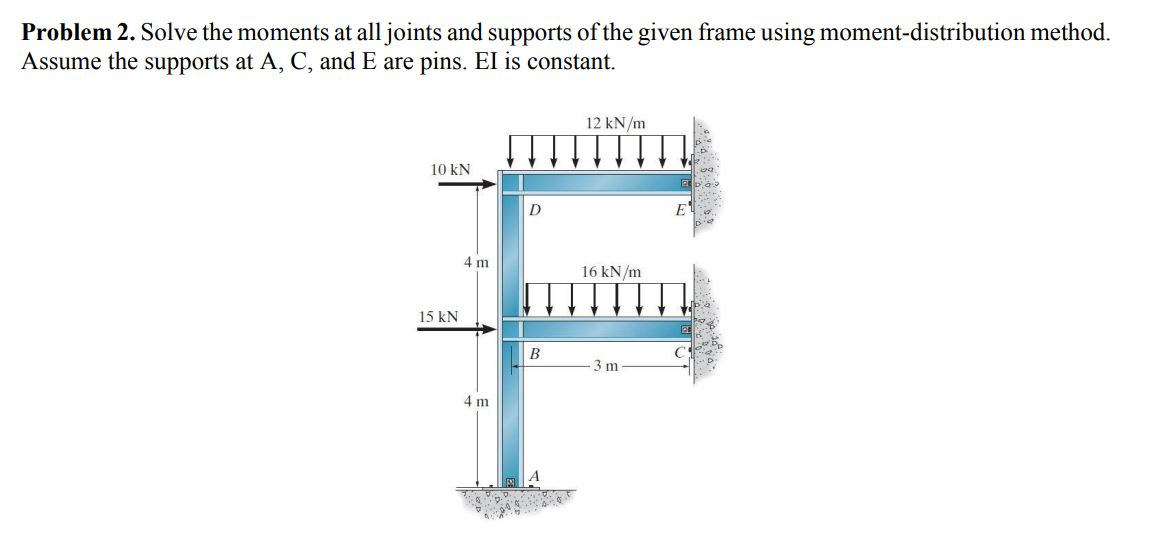Solve All joints and supports using moment distribution method! Problem 2. Solve the moments at all joints and supports of the given frame using moment-distribution method. Assume the supports at A, C, and E are pins. El is constant. 12 kN/m 10 KN D 4 m 16 kN/m 15 kN B 3 m 4 m

• ### determine the X component of reaction at A determine the Y component of reaction at A...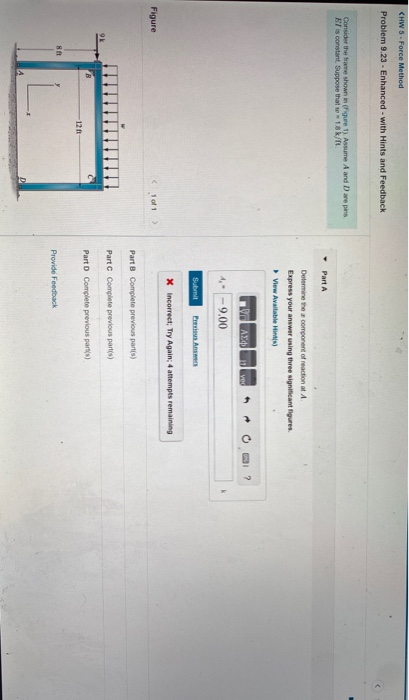determine the X component of reaction at A determine the Y component of reaction at A determine the Xcomponent of reaction at D determine the Y component of reaction at D CHW 5 -Force Method Problem 9.23 - Enhanced with Hints and Feedback Consider the trave shown in Figure 1). Assume A and Dare pins BT is constant Suppose that 1.8k/t. Part A Determine the component of reaction at Express your answer using three significant figures. View Available in ΑΣ...

• ### Statics. Method of sections Problem No.1 (25%): Using the method of sections determine the forces in...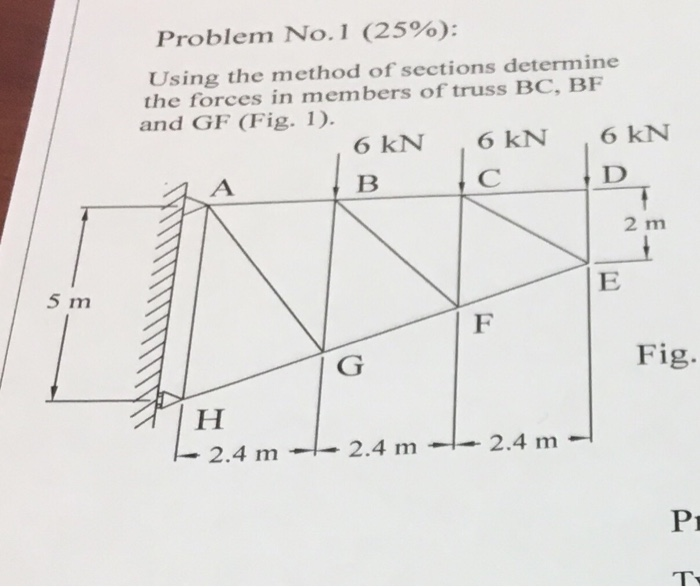Statics. Method of sections Problem No.1 (25%): Using the method of sections determine the forces in members of truss BC, BF and GF (Fig. 1). 6 KN 6 KN 6 KN B. С 2 m 5 m Fig. ІН 2.4 m - 2.4 m - 2.4 m Pi

• ### Using the stiffness method, determine the axial forces within members and the displacements of jo...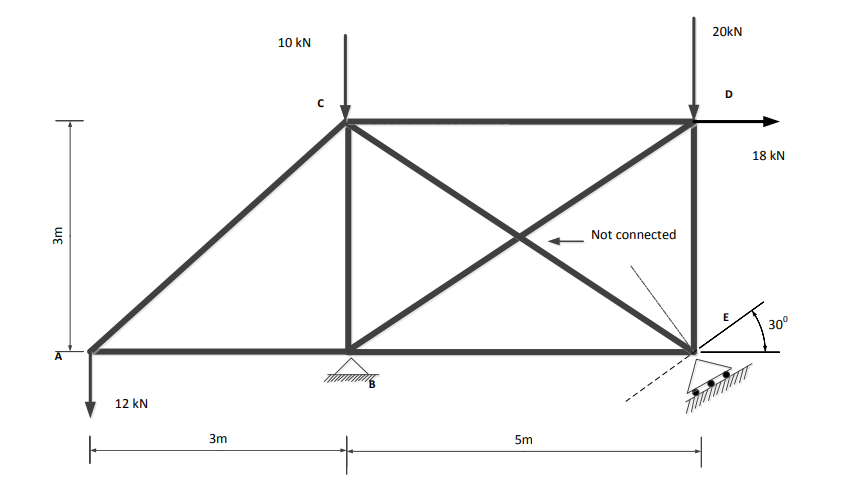Using the stiffness method, determine the axial forces within members and the displacements of joints of the truss shown in the Figure 1. The truss was built using 50 mm x 50 mm x 3 mm SHS with E= 200 GPa (approx). (Cross members BD and CE are not connected at the middle) (a) Show local stiffness matrices for each member and the assembled global stiffness matrix. Show your step by step solution. (30 Marks) (b) Use an appropriate method...

• ### compute the bending moments, shear forces and axial forces for the frame shown, using portal method....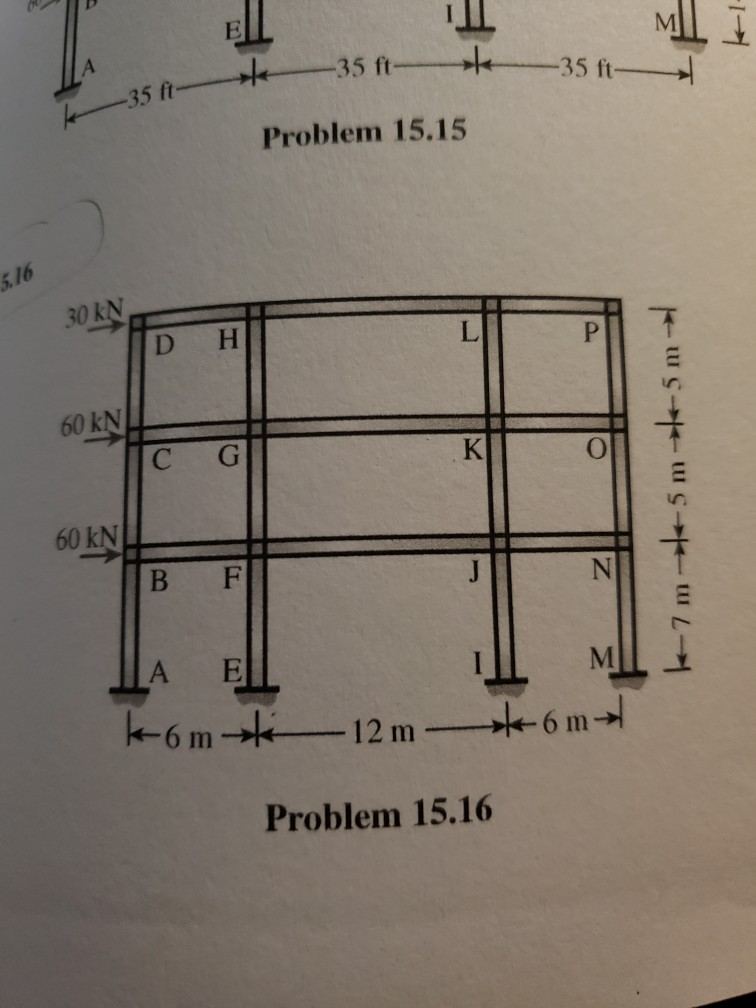compute the bending moments, shear forces and axial forces for the frame shown, using portal method. 3535 ft 35 ft Problem 15.15 5.16 30 kN D H 60 kN 0NG C G 60 kN Problem 15.16

• ### How to solve for reaction forces 5. Find the reaction forces at Point A and B....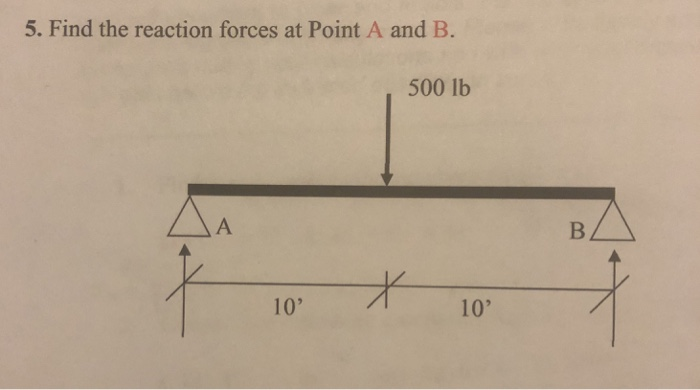How to solve for reaction forces 5. Find the reaction forces at Point A and B. 500 lb 10' 10'